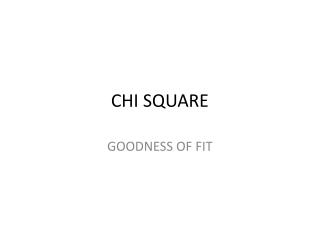DownloadDownload PresentationCHI SQUARE

# CHI SQUARE

Download Presentation## CHI SQUARE

- - - - - - - - - - - - - - - - - - - - - - - - - - - E N D - - - - - - - - - - - - - - - - - - - - - - - - - - -
##### Presentation Transcript

1. CHI SQUARE GOODNESS OF FIT

2. RESEARCH QUESTION • Is the proportion of number of students occupying the Emotional Intelligence level for moderate and high is equal?

3. HYPOTHESIS • Null hypothesis : n1 = n2 The number of students for both medium and high level of Emtional Intelligence is equal, where:n1 = the number students in the range of moderate Emotional Intelligence (EI)n2 = the number students in the range of high Emotional Intelligence (EI) • Alternative hypothesis: n1 ≠ n2The number of students for both moderate and high level of Emotional Intelligence (EI) is not the same.

4. TEST OF NORMALITIES BASED ON KOLMOGOROV-SMIRNOV • Since our subjects is more than 100, so we use Kolmogorov-Smirnov Normality Test. • From the table, for both moderate and high EI, the Sig. is 0.200 which is more than the α = 0.05. Therefore, the test is not statistically significant.

5. CHI-SQUARE TEST The case summary processing indicates that the data from 133 cases were included in these analyseswith 106 for moderate EI and 27 for high EI

6. GOODNESS OF FIT CROSSTABULATION • From the table, we can see that the observed N is unequal proportion for moderate and high EI

7. TEST STATISTIC • Asymp. Sig is less than 0.01 • So, Asymp. Sig is < 0.05 when compared to  (0.05), therefore we say that the observed frequencies is significantly different from the expected frequencies. • Note below informs us that 0% of categories had expected frequencies below five, and as it is less than 4454.5, therefore the expected frequencies assumption has not been violated.

8. CHI-SQUARE TEST FOR GOODNESS OF FIT:EFFECT SIZE • Effect size • w = √ X² N • w = √ 4150.697² 133 = 31.208 (this is a mediumeffect)

9. CONCLUSION • The CHI-SQUARE Goodness of Fit test (with α = 0.05) was used to evaluate whether the proportion of students with moderate EI and high EI of the biomedical students is equal or not. • The chi-square test was not statistically significant, p = 0.200. • Therefore, we do not accept Null hypothesis. • We can conclude that the proportion of students with moderate EI and high EI is not equal and this is proven by the test statistic with • Moderate EI = 7495 • High EI = 1414 • This show the unequal proportion of the number of students.